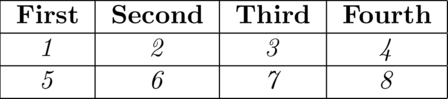# Latex tables with awk

## 03.02.2019

UnixLatex

There are numerous ways of doing tables in Latex. I guess most beginners start by manually inputting values inside \begin{table} and \end{table} but quickly realize how painful that is. The main methods I’ve used in the past are

Xtables, pgfplottables and pandas_to_latex are all great tools. However, it can sometimes be a bit of hassle to tame them to output exactly what you want. Additionally, they are a bit “bloaty” if you subscribe to the Unix philosophy.

So if we are going to be Unixy about this, the obvious tool for generating tables from csv files is awk. It is actually really simple and concise. Plus you get all the stdin and stdout piping goodness. Here’s one way of doing it (this is based on this stackoverflow answer regarding HTML tables).

#!/bin/awk -f
BEGIN {
FS=","; ORS = " "
print "\\begin{tabular}"
}
# Function to print a row with one argument to handle a textformat
function printRow(textformat) {
print "\t"
for(i=1; i<=NF; i++)    {
if (i < NF) print textformat"{"$i"}"" &" else print textformat"{"$i"}"
}
print "\\\\\\hline \n"
}
# If CSV file line number (NR variable) is 1
NR==1 {
printf ("{|")
for(c=0;c<NF;c++) printf "c|"; printf "}"
print ("\n\\hline\n")
printRow("\\textbf")
}
# If CSV file line number (NR variable) is greater than 1
NR>1 {
printRow("\\textit")
}
# Print footer
END {
print "\\end{tabular}"
}

So with a csv like this:

First,Second,Third,Fourth
1,2,3,4
5,6,7,8

You get something like this:

\begin{tabular} {|c|c|c|c|}
\hline
\textbf{First} & \textbf{Second} & \textbf{Third} & \textbf{Fourth} \\\hline
\textit{1} & \textit{2} & \textit{3} & \textit{4} \\\hline
\textit{5} & \textit{6} & \textit{7} & \textit{8} \\\hline
\end{tabular} 

which looks something like this:Of course this is a pretty simple looking table, but in my experience simple is usually better.